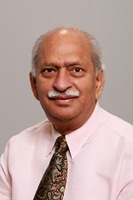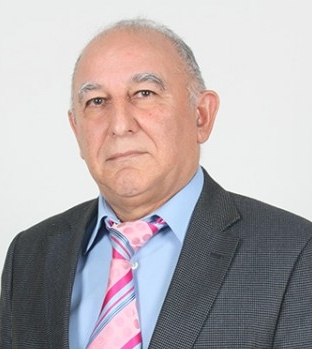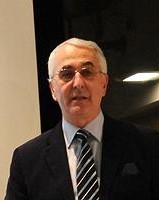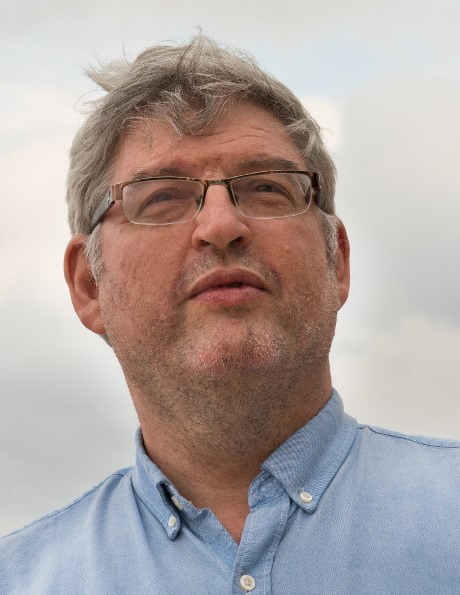### Invited SpeakersRavi P. Agarwal Texas A&M University-Kingsville, Texas, USA Title: Upper and lower solution method for nth order BVPs on an infinite interval Abstract: This work is devoted to study a nth order ordinary differential equation on a half-line with Sturm-Liouville boundary conditions. The existence results of a solution and triple solutions are established by employing a generalized version of the upper and lower solution method, Sch¨auder fixed point theorem, and topological degree theory. In our problem the nonlinearity depends on derivatives, and we allow solutions to be unbounded, which is an extra interesting feature. To demonstrate the usefulness of our results we illustrate two examples.Veli Shakhmurov Antalya Bilim University, Antalya, Turkey Title: The Lp regularity properties of nonlocal abstract evaluation equations and applications Abstract: In this talk, the Cauchy problem for linear and nonlinear nonlocal wave equations are studied.The equation involves convolution terms with a general kernel functions whose Fourier transform are operator functions defined in a Banach space E together with some growth conditions. Here, assuming enough smoothness on the initial data and the operator functions, the existence, unique- ness, regularity and blow up properties of solutions are established in terms of fractional powers of a given sectorial operator. We can obtain a different classes of nonlocal wave equations by choosing the space E and linear operators, which occur in a wide variety of physical systems.Vicentiu D. Radulescu University of Craiova, Romania & Institute of Mathematics of the Romanian Academy, Bucharest Title: Nonlinear equations with double phase: New phenomena and open problems Abstract: We report on some recent results concerning noticeable phenomena that arise in the study of double-phase equations with Dirichlet boundary condition. We first consider the isotropic setting described by the (p, q)-differential operator and we point out the following results: (i) a discontinuity property of the spectrum; (ii) the existence of a continuous spectrum that concentrates at infinity. Next, we are concerned with the anisotropic framework corresponding to a double-phase energy with several variable exponents. We study a class of non-standard problems with triple regime “subcritical-critical-supercritical”. Exotic phenomena of this type become possible due to the presence of variable exponents.We establish several existence properties corresponding to the radial or nonradial cases, respectively to a variable potential which is singular on the boundary.Patrick Guidotti Department of Mathematics, University of California, CA, USA Title: The SEEM Method Abstract: In this we shall discuss a novel numerical method to solve boundary value problems in complex domains that shares its basic idea with so-called fictitious domain methods and can be interpreted and analyzed by using the meshfree methods philosophy. It reduces the solution of a (initial) boundary value problem in general domains to an optimization problem for an extension of the solution to a larger, simpler, encompassing domain where the equations are considered as constraints. The simplicity of the encompassing domain is exploited in order to obtain simple and straightforward discretization. This, in particular, does not require the use nor generation of domain specific meshes.Ahmed Zayed Department of Mathematical Sciences, DePaul University, Chicago, Illinois, USA Title: On the coupled fractional fourier transform: Properties and extension Abstract: The Fourier transform, which has ubiquitous applications in mathematics, physics, and engineering, has been studied extensively for over a century. In 1980 a fractional version of the Fourier transform was introduced but did not received much attention until the early 1990s when it was found to have numerous applications in optics and time-frequency representation. The fractional Fourier transform, denoted by Fθ, depends on a parameter 0 ≤ θ ≤ π/2, so that when θ = 0, F0 is the identity transformation and when θ = π/2, Fπ/2 is the standard Fourier transform. In this talk we discuss a novel generalization of the fractional Fourier transform to two dimensions, which is called the coupled fractional Fourier transform and is denoted by Fα,β. This transform depends on two indepen- dent angles α and β, with 0 ≤ α, β ≤ π/2, so that F0,0 is the identity transformation and Fπ/2,π/2, is the two-dimensional Fourier transform. For other values of α and β, we obtain other interesting configurations of the transform. One immediate application of this transform is in time-frequency representation because of its close relationship to the Wigner distribution function. After a brief introduction to the coupled fractional Fourier transform, we shall discuss some of its properties and extensions to diff t functions spaces.Shmuel Weinberger Department of Mathematical Sciences, University of Chicago, Chicago, Illinois, USA Title: A coarse view of critical points. Abstract: Critical points of real valued functions are defined via their infinitesimal properties (i.e. using the first derivative to define them and the second derivative for their index); as a result, they can be highly unstable (with bad numerical implications). However, they are topologically significant as is reflected in the basic fact that any continuous function on a (locally connected) disconnected compact topological space has at least two local minima (which for smooth functions would be critical points of index 0). I will describe some large-scale or multi-scale ideas that are used in connecting geometry and critical point theory which can be traced back to Weierstrass's nowhere differentiable function and the classical work of Chebyshev in approximation theory, and have been applied to Riemannian geometry, Topological Data Analysis and Hamiltonian dynamics.